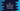# C# program to find the sum of odd and even numbers in an array## How to find the sum of odd and even numbers in an array in C#:

In this post, we will learn how to find the sum of all odd and even numbers in an array in C#. This program will create the array by taking the inputs from the user and then it will find the sum.

To build the array, we need to use one loop. This loop will ask the user to enter the numbers one by one and it will insert them to an array. It will again use another loop to find the sum of odd and even numbers in the user given array.

The loop will iterate through the numbers one by one, it will check if the current value is odd or even and it will add it to a sum variable.

We will create two sum variables. One for the sum of odd numbers and another for the even numbers. These variables will be initialized to 0. Once we keep adding the values, at the end of the loop, these variables will hold the total sum.

### C# program:

Let’s take a look at the below program:

``````using System;
class Program {
static void Main() {
int size;
int oddSum = 0, evenSum = 0;

Console.WriteLine("Enter the size of the array:");

int[] arr = new int[size];

Console.WriteLine("Enter the array values:");
for(int i = 0; i< size; i++){
}

for(int i = 0; i< size; i++){
if(arr[i] % 2 == 0){
evenSum += arr[i];
}else{
oddSum += arr[i];
}
}

Console.WriteLine("Even Sum: "+evenSum+" Odd Sum: "+oddSum);
}
}``````

Here,

• size variable is used to hold the size of the array. oddSum is to hold the sum of all odd numbers in the array and evenSum to hold the sum of all even numbers.
• It is asking the user to enter the size of the array and storing it in size by reading the user-input value.
• arr is an array of integers. It is created by using the user-input size.
• The first for loop is used to read the user input values for the array. It runs for i = 0 to i = size - 1. It reads all values from the user and inserts them to the array arr.
• The second for loop also iterates through the values in the array. For each value, it checks if it is odd or even. If it is even, it adds that value to evenSum. Else, it adds that value to oddSum.
• Finally, after the loop ends, it prints these sum values evenSum and oddSum.

It will print the below output:

``````Enter the size of the array:
5
Enter the array values:
1
2
3
4
5
Even Sum: 6 Odd Sum: 9``````

### By using a foreach loop:

We can also write this program with a different loop like foreach. Let’s take a look at the below program:

``````using System;
class Program {
static void Main() {
int size;
int oddSum = 0, evenSum = 0;

Console.WriteLine("Enter the size of the array:");

int[] arr = new int[size];

Console.WriteLine("Enter the array values:");
for(int i = 0; i< size; i++){
}

foreach(int value in arr){
if(value % 2 == 0){
evenSum += value;
}else{
oddSum += value;
}
}

Console.WriteLine("Even Sum: "+evenSum+" OddSum: "+oddSum);

}
}``````

Here,

• We replaced the second for loop with foreach. It is working in a same way, but if we use foreach, we don’t have to access the numbers using index. We can directly get its value.

If you run this program, it will also print similar outputs.